# Excel Functions

Microsoft Excel provides many built-in functions that we can use to perform specific calculations or to return information about the spreadsheet data.

The easy way of inputting an Excel Function is to simply type the function directly into an Excel cell, with its arguments enclosed in brackets.

For a beginner, or for writing a complex formula, we may find it easier to use the Function Arguments dialog box, which helps you to input functions and formulas more easily.

Using the Function Arguments Dialog Box

The 'Function Arguments' dialog box automatically pops up when you select an Excel function using one of the following methods:

• Method 1: Select the Insert Function button (denoted by the ƒx symbol) from the left side of the formula bar, and then select a function name from the 'Insert Function' dialog box.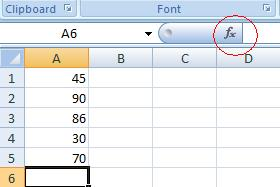• Method 2: Select a function from one of the lists in the 'Function Library' group, on the Formulas tab of the Excel ribbon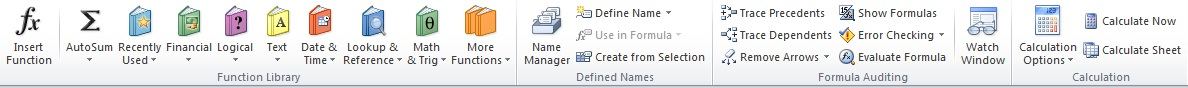When you select a function using one of the methods above, Excel automatically displays the 'Function Arguments' dialog box to assist you in inputting your selected function.

The Excel Functions are organised into various categories ( Text, Logical, Date and Time etc ) to help you to locate the funtions which we need from the Excel Categories.

The Excel Functions Categories are as follows
• Text Functions
• Logical Functions
• Information Functions
• Date and Time Functions
• Lookup and Reference Functions
• Math and Trig Functions
• Statistical Functions
• Database Functions
• Financial Functions
• Engineering Functions
• Cube Functions
• Web Functions
The most basic functions normally used are Sum(), Count(), Max(), Min() and the logical IF() funtion.

## SUM

The SUM function is the first must-know formula in Excel. It usually aggregates values from a selection of columns or rows from your selected range.

=SUM(number1, [number2], …)

Example:

=SUM(C4:G4) – A simple selection that sums the values of a row.

=SUM(A1:A5) – A simple selection that sums the values of a column.

=SUM(A2:A7, A9, A12:A15) – A sophisticated collection that sums values from range A2 to A7, skips A8, adds A9, jumps A10 and A11, then finally adds from A12 to A15.

=SUM(A1:A5)/20 – Shows you can also turn your function into a formula.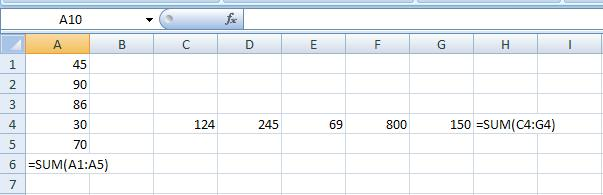## COUNT

The COUNT function counts all cells in a given range that contain only numeric values.

=COUNT(value1, [value2], …)

Example:

COUNT(A:A) – Counts all values that are numerical in A column. However, you must adjust the range inside the formula to count rows.

COUNT(A1:A5) – Now it can count rows.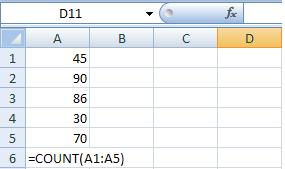## AVERAGE

The AVERAGE function should remind you of simple averages of data such as the average number of shareholders in a given shareholding pool.

=AVERAGE(number1, [number2], …)

Example:

=AVERAGE(A1:A10) – Shows a simple average, also similar to (SUM(A1:A10)/10)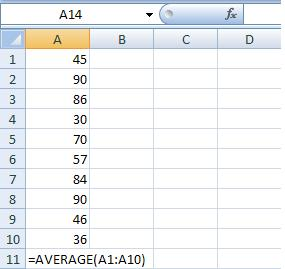## MAX & MIN

The MAX and MIN functions help in finding the maximum number and the minimum number in a range of values.

=MAX(number1, [number2], …)

=MIN(number1, [number2], …)

Example:

=MAX(A1:B10) – Similarly, it finds the maximum number between column A from A1 and column B from B1 to row 10 in both columns A and B.

=MIN(A1:B10) – Similarly, it finds the minimum number between column A from A1 and column B from B1 to row 10 in both columns A and B.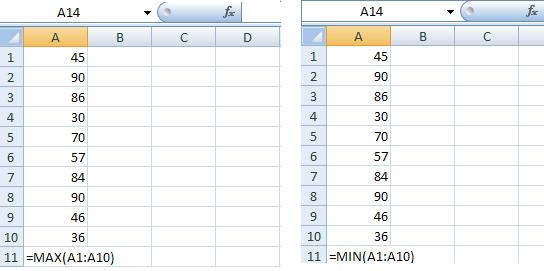## IF

The IF logical function is often used when you want to sort your data according to a given logic. The best part of the IF formula is that you can embed formulas and function in it.

=IF(logical_test, [value_if_true], [value_if_false])

Example:

=IF(A1<35, ‘Fail,’ ‘Pass’) – Checks if the value at A1 is less than the 35. If the logic is true, let the cell value be Fail, else, Pass

=IF(SUM(C1:C10) > SUM(D1:D10), SUM(C1:C10), SUM(D1:D10)) – An example of a complex IF logic. First, it sums C1 to C10 and D1 to D10, then it compares the sum. If the sum of C1 to C10 is greater than the sum of D1 to D10, then it makes the value of a cell equal to the sum of C1 to C10. Otherwise, it makes it the SUM of C1 to C10.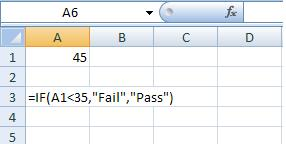To Learn Advanced Excel Commands like Sparkline / Data Validation Whatapp/Call 8422000829. Disount Code WEBSAB501

Home Chapter 1 Chapter 2 Chapter 3 Chapter 4 Chapter 5 Chapter 7 Chapter 8 Chapter 9 Chapter 10 Excel Shortcuts

Keep Learning...